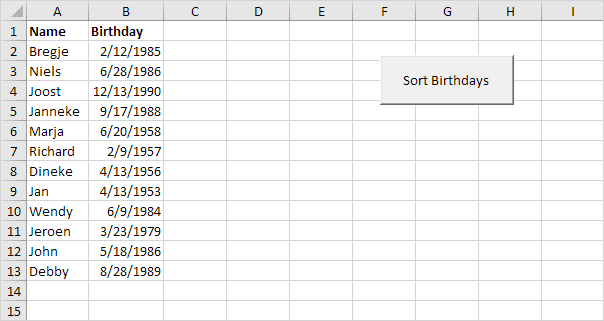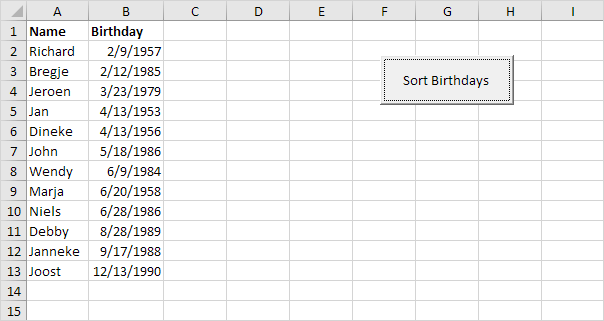# Sort Birthdays

Below we will look at a program in Excel VBA that sorts birthdays by months first and days second (so we ignore years). We want the birthday with the lowest month number at the first position. If there are birthdays with equal month numbers, we want the birthday with the lowest day number first. Are you ready?

Situation:Note: Dates are in US Format. Months first, Days second. This type of format depends on your windows regional settings.

1. First, we declare eight variables. One Date variable we call tempDate, one String variable we call tempName. The other six variables are Integer variables with names monthToCheck, dayToCheck, monthNext, dayNext, i and j.

Dim tempDate As Date, tempName As String
Dim monthToCheck As Integer, dayToCheck As Integer, monthNext As Integer, dayNext As Integer, i As Integer, j As Integer

2. We start two For Next loops.

For i = 2 To 13
For j = i + 1 To 13

Example: for i = 2, j = 3, 4, ... , 12 and 13 are checked.

3. We initialize four Integer variables. We use the Month function to get the month of a date and the Day function to get the day of a date.

monthToCheck = month(Cells(i, 2).Value)
dayToCheck = day(Cells(i, 2).Value)

monthNext = month(Cells(j, 2).Value)
dayNext = day(Cells(j, 2).Value)

For example: at the start, for i = 2; the date of Bregje, and j = i + 1 = 2 + 1 = 3; the date of Niels will be chosen.

4. To sort the dates properly, we compare the first date (monthToCheck and dayToCheck) with the next date (monthNext and dayNext). If the next date is 'lower', we swap the dates and names. Add the following If Then statement.

If (monthNext < monthToCheck) Or (monthNext = monthToCheck And dayNext < dayToCheck) Then

End If

If the above statement is true, we swap the dates and names.

For example: for i = 2 and j = 3, the date of Bregje and Niels are checked. MonthNext = 6, monthToCheck = 2. The above statement is not true since monthNext is higher than monthToCheck. Excel VBA increments j by 1 and repeats the code lines for i = 2 and j = 4. You can easily see that Joost (j = 4) has a higher month number than Bregje, so we go to the next one. We get the same result for j = 5 and j = 6. When we arrive at j = 7, we have the following variables: monthNext = 2 and dayNext = 9. MonthToCheck = 2 and dayToCheck = 12. Now the above statement is true since monthNext = monthToCheck and dayNext (9) is lower than dayToCheck (12).

5. We swap the dates. We temporarily store one date to tempDate, so that Excel VBA can swap the dates properly. Add the following code lines in the If statement.

'swap dates
tempDate = Cells(i, 2).Value
Cells(i, 2).Value = Cells(j, 2).Value
Cells(j, 2).Value = tempDate

6. We do the same with the names. Add the following code lines in the If statement.

'swap names
tempName = Cells(i, 1).Value
Cells(i, 1).Value = Cells(j, 1).Value
Cells(j, 1).Value = tempName

7. We close the second For Next loop (Outside the If statement).

Next j

For i = 2 and j = 7, Excel VBA swapped the dates and names. That means we get Richard at the first position and Bregje at position 7. That also means we get a new monthToCheck and dayToCheck at the start of our next iteration (for i = 2 and j = 8). We will now compare Richard with Dineke (j = 8). You can easily see that there is no need to replace those dates and names because Richard has a 'lower' date. As a matter of fact, there is no need to replace Richard (i = 2) with Jan (j = 9), Wendy (j = 10), Jeroen (j = 11), John ( j= 12) and Debby (j = 13). That is because Richard has the 'lowest' date. This way Excel VBA will get (for i = 2) the 'lowest' date at the first position. To get the second 'lowest' date at the second position, Excel VBA repeats the exact same steps for i = 3. To get the third 'lowest' date at the third position, Excel VBA repeats the exact same steps for i = 4, etc.

8. Close the first For Next loop (Outside the If statement).

Next i